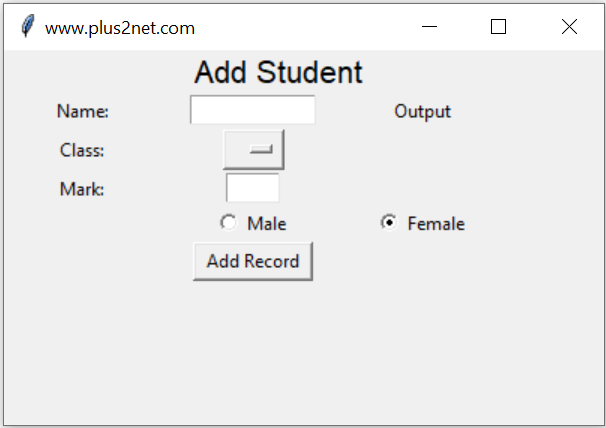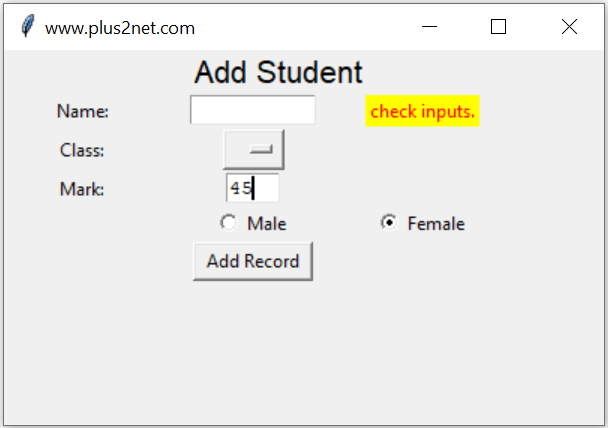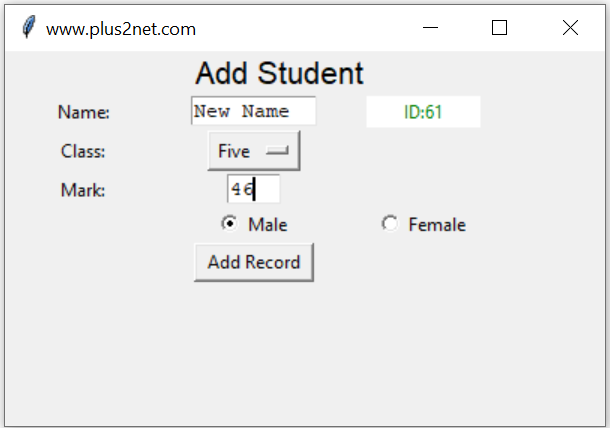# Inserting Data to sqlite table

Basics of Python Tkinter

Add records to sqlite Student table by taking user entered inputs from a Tkinter window.There are three parts in this script.
1. Part 1: Create the GUI window using tkinter.
2. Part 2: Validate the user entered data.
3. Part 3: Add data to sqlite table.

## Part 1 : Tkinter window to accept user inputs

User will enter four input data using our Tkinter window. Main components are here

Name : String of name through a text box t1
Class : Select one option from the dropdown OptionMenu
Mark : Integer value ( number ) through text box t3
Sex : Selection through a pair of radio buttons ( Male , Female or other )
Submit : Button to trigger a click event to handle all the inputs.

There are Labels to show message about the input data ( l1,l2,l3 ) and one final Label ( l5 ) to display the output or return id of the record after inserting the data in table. One OptionMenu ( opt1) is used for selection of class.
``````l0 = tk.Label(my_w,  text='Add Student',
font=('Helvetica', 16), width=30,anchor="c" )
l0.grid(row=1,column=1,columnspan=4)

l1 = tk.Label(my_w,  text='Name: ', width=10,anchor="c" )
l1.grid(row=3,column=1)

t1 = tk.Text(my_w,  height=1, width=10,bg='white')
t1.grid(row=3,column=2)

l2 = tk.Label(my_w,  text='Class: ', width=10 )
l2.grid(row=4,column=1)

# add list box for selection of class
options = StringVar(my_w)
options.set("") # default value

opt1 = OptionMenu(my_w, options, "Three", "Four", "Five")
opt1.grid(row=4,column=2)

l3 = tk.Label(my_w,  text='Mark: ', width=10 )
l3.grid(row=5,column=1)

t3 = tk.Text(my_w,  height=1, width=4,bg='white')
t3.grid(row=5,column=2)

r1.grid(row=6,column=2)

r2.grid(row=6,column=3)

b1 = tk.Button(my_w,  text='Add Record', width=10,
b1.grid(row=7,column=2)

my_str = tk.StringVar()

l5 = tk.Label(my_w,  textvariable=my_str, width=10 )
l5.grid(row=3,column=3)
my_str.set("Output")``````
The Button b1 is used to execute the function add_data() by using the command or click event of the button.
``````b1 = tk.Button(my_w,  text='Add Record', width=10,
b1.grid(row=7,column=2)``````
Inside the function add_data() we have two sections. In first section we will check each input data. It is expected that no input is left blank by user. Without filling the details if user has clicked the button ( b1 ) then we will display a message through our Label ( l5 ) asking to check the inputs ( Read the else part of the if-else).
``````def add_data():
flag_validation=True # set the flag
# length of my_name , my_class and my_gender more than 2
if(len(my_name) < 2 or len(my_class)<2  or len(my_gender) < 2 ):
flag_validation=False
try:
val = int(my_mark) # checking mark as integer
except:
flag_validation=False

if(flag_validation):
#  Part 3  database insert part
else:
l5.config(fg='red')   # foreground color
l5.config(bg='yellow') # background color
my_str.set("check inputs.")``````In this code we first set one flag validation ( flag_validation ) to True, if any where the validation of the inputs fails then we will change this status of validation to False. ( this is part of the above code )
``````if(len(my_name) < 2 or len(my_class)<2  or len(my_gender) < 2 ):
flag_validation=False
try:
val = int(my_mark) # checking mark as integer
except:
flag_validation=False ``````
After all the checks ( validation of inputs ) if the flag ( flag_validation ) is True , then we can start adding the data to sqlite database student table. This completes the Part 2 and in next section we will add data to our table in Part 3 .

## Database connection

Connect to database by using the sqlite3 .
``````import sqlite3
my_conn = sqlite3.connect('my_db.db')``````
We used Parameterized query to add one record to student table. The INSERT Query is used to add the record to sqlite database.
``````my_data=(None,my_name,my_class,my_mark,my_gender)
my_query="INSERT INTO student values(?,?,?,?,?)"
my_conn.execute(my_query,my_data)
my_conn.commit()``````
Our student table has one Primary Key as ID column. So after adding the record we can collect the unique id of this primary key and display it to user to show successful completion of adding record. Here last_insert_rowid() stores the value of this new ID.
``````	    x=my_conn.execute('''select last_insert_rowid()''')
id=x.fetchone()
l5.grid()
l5.config(fg='green') # foreground color
l5.config(bg='white') # background color
my_str.set("ID:" + str(id))
l5.after(3000, lambda: l5.grid_remove() )``````We have used the Label l5 to display the id of the record added. This display of Id ensures that the record is inserted successfully.

We want to show the ID ( after adding the record ) or the error message for some time and it should vanish after the time delay. In above code the last line does that for us. Learn more on how to add or remove the widget in a grid here.
``l5.after(3000, lambda: l5.grid_remove() )``
Remove the user entered text after adding of records
``````	t1.delete('1.0',END)  # reset the text entry box
t3.delete('1.0',END)  # reset the text entry box``````
``````	l5.config(fg='green') # foreground color
l5.config(bg='white') # background color
my_str.set("ID:" + str(id.lastrowid))``````
The full code is here.
``````# www.plus2net.com
import sqlite3
my_conn = sqlite3.connect('my_db.db')
#print("Opened database successfully");
import tkinter  as tk
from tkinter import *
my_w = tk.Tk()
my_w.geometry("400x250")

my_w.title("www.plus2net.com")
font=('Helvetica', 16), width=30,anchor="c" )
l0.grid(row=1,column=1,columnspan=4)

l1 = tk.Label(my_w,  text='Name: ', width=10,anchor="c" )
l1.grid(row=3,column=1)

t1 = tk.Text(my_w,  height=1, width=10,bg='white')
t1.grid(row=3,column=2)

l2 = tk.Label(my_w,  text='Class: ', width=10 )
l2.grid(row=4,column=1)

# add list box for selection of class
options = StringVar(my_w)
options.set("") # default value

opt1 = OptionMenu(my_w, options, "Three", "Four", "Five")
opt1.grid(row=4,column=2)

l3 = tk.Label(my_w,  text='Mark: ', width=10 )
l3.grid(row=5,column=1)

t3 = tk.Text(my_w,  height=1, width=4,bg='white')
t3.grid(row=5,column=2)

r1.grid(row=6,column=2)

r2.grid(row=6,column=3)

b1 = tk.Button(my_w,  text='Add Record', width=10,
b1.grid(row=7,column=2)

my_str = tk.StringVar()
l5 = tk.Label(my_w,  textvariable=my_str, width=10 )
l5.grid(row=3,column=3)
my_str.set("Output")
flag_validation=True # set the flag
# length of my_name , my_class and my_gender more than 2
if(len(my_name) < 2 or len(my_class)<2  or len(my_gender) < 2 ):
flag_validation=False
try:
val = int(my_mark) # checking mark as integer
except:
flag_validation=False

if(flag_validation):
try:
#print("Connected to database successfully")
my_data=(None,my_name,my_class,my_mark,my_gender)
my_query="INSERT INTO student values(?,?,?,?,?)"
my_conn.execute(my_query,my_data)
my_conn.commit()
x=my_conn.execute('''select last_insert_rowid()''')
id=x.fetchone()
l5.grid()
l5.config(fg='green') # foreground color
l5.config(bg='white') # background color
my_str.set("ID:" + str(id))
l5.after(3000, lambda: l5.grid_remove() )
t1.delete('1.0',END)  # reset the text entry box
t3.delete('1.0',END)  # reset the text entry box

except sqlite3.Error as my_error:
l5.grid()
#return error
l5.config(fg='red')   # foreground color
l5.config(bg='yellow') # background color
print(my_error)
my_str.set(my_error)
else:
l5.grid()
l5.config(fg='red')   # foreground color
l5.config(bg='yellow') # background color
my_str.set("check inputs.")
l5.after(3000, lambda: l5.grid_remove() )
my_w.mainloop()

my_conn.close()``````

Subscribe to our YouTube Channel here

## Subscribe

* indicates required
Subscribe to plus2netplus2net.com